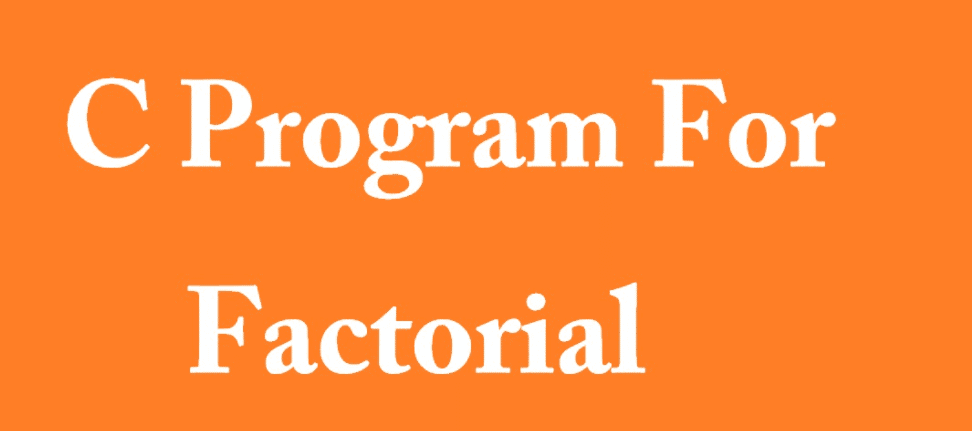# C program to find factorial of user given number – C programming guide

## C program to find factorial of user given number – for beginner

C program to find factorial | Let’s assume, Factorial of a positive number ‘n’ given by user

factorial of n(n!) = 1 *  2 * 3 * ……. upto Nth number.

Factorial of negative number is not possible. In simple word it doesn’t exists. ok lets start our program:

### Find factorial of a user given number using C language.

```#include<stdio.h>
#include <conio.h>
main(){
int num, i, fact=1;
printf("Enter a number");
scanf("%d",&num);
for(i=num;i>=0;i--){
fact = fact*i;
}
printf("Factorial of %d is %d", num,fact);
getch();
}
```

Ascending and Descending order using C programming language

#### C program to find factorial of given number

```#include<stdio.h>
#include<conio.h>
void getFactorial(int,int *); //function
int main(){
int i,factorial,n;

printf("Enter a number: ");
scanf("%d",&n);

getFactorial(n,&factorial);
printf("Factorial of %d is: %d",n,*factorial);

return 0;
}

void getFactorial(int n,int *factorial){
int i;

*factorial =1;

for(i=1;i<=n;i++)
*factorial=*factorial*i;
}```

C documentation